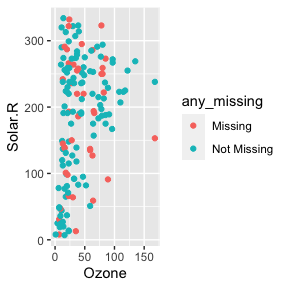# Exploring Imputed Values

#### 2020-09-02

Imputating missing values is an iterative process. naniar aims to make it easier to manage imputed values by providing the nabular data structure to simplify managing missingness. This vignette provides some useful recipes for imputing and exploring imputed data.

naniar implements a few imputation methods to facilitate exploration and visualisations, which were not otherwise available: impute_below, and impute_mean. For single imputation, the R package simputation works very well with naniar, and provides the main example given.

# Using impute_below

impute_below imputes values below the minimum of the data, with some noise to reduce overplotting. The amount data is imputed below, and the amount of jitter, can be changed by changing the arguments prop_below and jitter.

library(dplyr)
#>
#> Attaching package: 'dplyr'
#> The following objects are masked from 'package:stats':
#>
#>     filter, lag
#> The following objects are masked from 'package:base':
#>
#>     intersect, setdiff, setequal, union
library(naniar)

airquality %>%
impute_below_at(vars(Ozone)) %>%
select(Ozone, Solar.R) %>%
#>       Ozone Solar.R
#> 1  41.00000     190
#> 2  36.00000     118
#> 3  12.00000     149
#> 4  18.00000     313
#> 5 -19.72321      NA
#> 6  28.00000      NA

# Using impute_mean

The mean can be imputed using impute_mean, and is useful to explore structure in missingness, but are not recommended for use in analysis. Similar to simputation, each impute_ function returns the data with values imputed.

Imputation functions in naniar implement “scoped variants” for imputation: _all, _at and _if.

This means:

• _all operates on all columns
• _at operates on specific columns, and
• _if operates on columns that meet some condition (such as is.numeric or is.character).

If the impute_ functions are used as-is - e.g., impute_mean, this will work on a single vector, but not a data.frame.

Some examples for impute_mean are now given:


impute_mean(oceanbuoys$air_temp_c) %>% head() #>  27.15 27.02 27.00 26.93 26.84 26.94 impute_mean_at(oceanbuoys, .vars = vars(air_temp_c)) %>% head() #> # A tibble: 6 x 8 #> year latitude longitude sea_temp_c air_temp_c humidity wind_ew wind_ns #> <dbl> <dbl> <dbl> <dbl> <dbl> <dbl> <dbl> <dbl> #> 1 1997 0 -110 27.6 27.1 79.6 -6.40 5.40 #> 2 1997 0 -110 27.5 27.0 75.8 -5.30 5.30 #> 3 1997 0 -110 27.6 27 76.5 -5.10 4.5 #> 4 1997 0 -110 27.6 26.9 76.2 -4.90 2.5 #> 5 1997 0 -110 27.6 26.8 76.4 -3.5 4.10 #> 6 1997 0 -110 27.8 26.9 76.7 -4.40 1.60 impute_mean_if(oceanbuoys, .predicate = is.integer) %>% head() #> # A tibble: 6 x 8 #> year latitude longitude sea_temp_c air_temp_c humidity wind_ew wind_ns #> <dbl> <dbl> <dbl> <dbl> <dbl> <dbl> <dbl> <dbl> #> 1 1997 0 -110 27.6 27.1 79.6 -6.40 5.40 #> 2 1997 0 -110 27.5 27.0 75.8 -5.30 5.30 #> 3 1997 0 -110 27.6 27 76.5 -5.10 4.5 #> 4 1997 0 -110 27.6 26.9 76.2 -4.90 2.5 #> 5 1997 0 -110 27.6 26.8 76.4 -3.5 4.10 #> 6 1997 0 -110 27.8 26.9 76.7 -4.40 1.60 impute_mean_all(oceanbuoys) %>% head() #> # A tibble: 6 x 8 #> year latitude longitude sea_temp_c air_temp_c humidity wind_ew wind_ns #> <dbl> <dbl> <dbl> <dbl> <dbl> <dbl> <dbl> <dbl> #> 1 1997 0 -110 27.6 27.1 79.6 -6.40 5.40 #> 2 1997 0 -110 27.5 27.0 75.8 -5.30 5.30 #> 3 1997 0 -110 27.6 27 76.5 -5.10 4.5 #> 4 1997 0 -110 27.6 26.9 76.2 -4.90 2.5 #> 5 1997 0 -110 27.6 26.8 76.4 -3.5 4.10 #> 6 1997 0 -110 27.8 26.9 76.7 -4.40 1.60 When we impute data like this, we cannot identify where the imputed values are - we need to track them. We can track the imputed values using the nabular format of the data. ### Track imputed values using nabular data We can track the missing values by combining the verbs bind_shadow, impute_, add_label_shadow. We can then refer to missing values by their shadow variable, _NA. The add_label_shadow function adds an additional column called any_missing, which tells us if any observation has a missing value. #### Imputing values using simputation We can impute the data using the easy-to-use simputation package, and then track the missingness using bind_shadow and add_label_shadow:  library(simputation) #> #> Attaching package: 'simputation' #> The following object is masked from 'package:naniar': #> #> impute_median ocean_imp <- oceanbuoys %>% bind_shadow() %>% impute_lm(air_temp_c ~ wind_ew + wind_ns) %>% impute_lm(humidity ~ wind_ew + wind_ns) %>% impute_lm(sea_temp_c ~ wind_ew + wind_ns) %>% add_label_shadow() We can then show the previously missing (now imputed!) data in a scatterplot with ggplot2 by setting the color aesthetic in ggplot to any_missing: library(ggplot2) ggplot(ocean_imp, aes(x = air_temp_c, y = humidity, color = any_missing)) + geom_point() + scale_color_brewer(palette = "Dark2") + theme(legend.position = "bottom")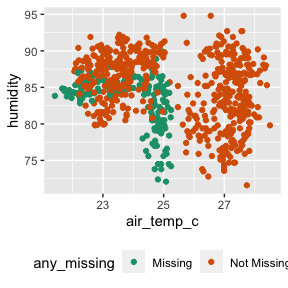Or, if you want to look at one variable, you can look at a density plot of one variable, using fill = any_missing  ggplot(ocean_imp, aes(x = air_temp_c, fill = any_missing)) + geom_density(alpha = 0.3) + scale_fill_brewer(palette = "Dark2") + theme(legend.position = "bottom") ggplot(ocean_imp, aes(x = humidity, fill = any_missing)) + geom_density(alpha = 0.3) + scale_fill_brewer(palette = "Dark2") + theme(legend.position = "bottom")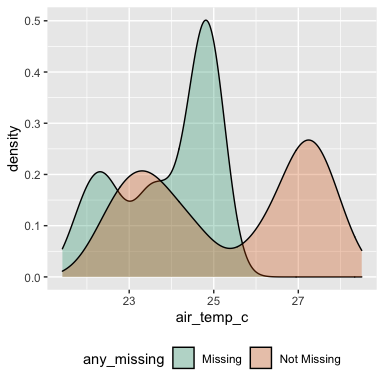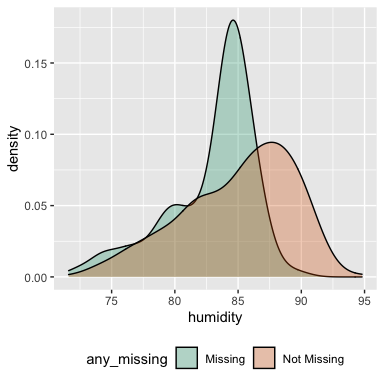We can also compare imputed values to complete cases by grouping by any_missing, and summarising. ocean_imp %>% group_by(any_missing) %>% summarise_at(.vars = vars(air_temp_c), .funs = funs(min, mean, median, max, .args = list(na.rm = TRUE))) #> Warning: funs() is deprecated as of dplyr 0.8.0. #> Please use a list of either functions or lambdas: #> #> # Simple named list: #> list(mean = mean, median = median) #> #> # Auto named with tibble::lst(): #> tibble::lst(mean, median) #> #> # Using lambdas #> list(~ mean(., trim = .2), ~ median(., na.rm = TRUE)) #> This warning is displayed once every 8 hours. #> Call lifecycle::last_warnings() to see where this warning was generated. #> # A tibble: 2 x 5 #> any_missing min mean median max #> <chr> <dbl> <dbl> <dbl> <dbl> #> 1 Missing 21.4 23.9 24.4 25.2 #> 2 Not Missing 22.1 25.3 25.8 28.5 # Improving imputations One thing that we notice with our imputations are that they aren’t very good - we can improve upon the imputation by including the variables year and latitude and longitude: ocean_imp_yr <- oceanbuoys %>% bind_shadow() %>% impute_lm(air_temp_c ~ wind_ew + wind_ns + year + longitude + latitude) %>% impute_lm(humidity ~ wind_ew + wind_ns + year + longitude + latitude) %>% impute_lm(sea_temp_c ~ wind_ew + wind_ns + year + longitude + latitude) %>% add_label_shadow() ggplot(ocean_imp_yr, aes(x = air_temp_c, y = humidity, color = any_missing)) + geom_point() + scale_color_brewer(palette = "Dark2") + theme(legend.position = "bottom")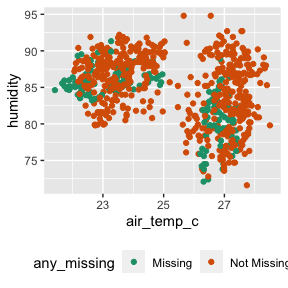# Other imputation approaches Not all imputation packages return data in tidy ## Hmisc aregImpute We can explore using a single imputation of Hmisc::aregImpute(), which allows for multiple imputation with bootstrapping, additive regression, and predictive mean matching. We are going to explore predicting mean matching, and single imputation.  library(Hmisc) #> Loading required package: lattice #> Loading required package: survival #> Loading required package: Formula #> #> Attaching package: 'Hmisc' #> The following object is masked from 'package:simputation': #> #> impute #> The following objects are masked from 'package:dplyr': #> #> src, summarize #> The following objects are masked from 'package:base': #> #> format.pval, units aq_imp <- aregImpute(~Ozone + Temp + Wind + Solar.R, n.impute = 1, type = "pmm", data = airquality) #> Iteration 1 Iteration 2 Iteration 3 Iteration 4 aq_imp #> #> Multiple Imputation using Bootstrap and PMM #> #> aregImpute(formula = ~Ozone + Temp + Wind + Solar.R, data = airquality, #> n.impute = 1, type = "pmm") #> #> n: 153 p: 4 Imputations: 1 nk: 3 #> #> Number of NAs: #> Ozone Temp Wind Solar.R #> 37 0 0 7 #> #> type d.f. #> Ozone s 2 #> Temp s 2 #> Wind s 2 #> Solar.R s 1 #> #> Transformation of Target Variables Forced to be Linear #> #> R-squares for Predicting Non-Missing Values for Each Variable #> Using Last Imputations of Predictors #> Ozone Solar.R #> 0.667 0.224 We are now going to get our data into nabular form, and then insert the imputed values:  # nabular form! aq_nab <- nabular(airquality) %>% add_label_shadow() # insert imputed values aq_nab$Ozone[is.na(aq_nab$Ozone)] <- aq_imp$imputed$Ozone aq_nab$Solar.R[is.na(aq_nab$Solar.R)] <- aq_imp$imputed\$Solar.R

In the future there will be a more concise way to insert these imputed values into data, but for the moment the method above is what I would recommend for single imputation.

We can then explore the imputed values like so:


ggplot(aq_nab,
aes(x = Ozone,
y = Solar.R,
colour = any_missing)) +
geom_point()